# Summary of Chapter 7:Energy

Kinetic Energy The kinetic energy of a particle is given by:where m is the particle's mass and v is the particles velocity.

Work Work is energy transfered to or from an object by a force. Energy transtered to an object is posative work and energy transfered from an object is negative work. Work done by a constant force (F) over displacement (d) is given by: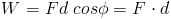Where the cosine of the angle between F and d is taken. If two forces act on one particle, the net work is equal to the work of each force combined, or the work done by the net force.

Work and Kinetic Energy Work is equal to the change in kinetic energy: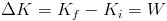Work done by Gravity Work done by gravity is given by: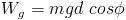where m is the mass of the particle, g is 9.8, d is distance and the cosine of the angle between Fg and d is taken.

Work Done Against Gravity when lifting or lowering an object, the work done is euqal to:If Ki and Kf are equal then this reduces to: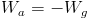Spring Force The force applied by a spring is equal to: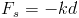where k is a constant, and d is the distance from the equilibrium point which the spring is stretched. Therefore, the spring force is a variable force.

Work Done by a Spring Force The work done by a spring on an object attached to the end of the spring is given by: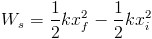If xi is equal to zero, then this reduces to:Work by a Variable Force Work is the area under a Force vs. Position graph: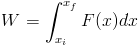Power Power due to a force is the rate of change at which that force does work on an object. if the force does work during a time interval Δt then the average power over that time interval is: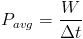For a force at an angle to the direction of travel (instantanious velocity v), the instantaneous power is equal to:Return to top
All information and equations are taken from and credited to Jearl Walker's Fundamental Physics.